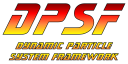#DPSFby Daniel Schroeder<PackageReference Include="DPSF" Version="2.5.0" />

# Orientation3D

public class Orientation3D
Class to hold and update an object's 3D Orientation, Rotational Velocity, and Rotational Acceleration.
public Quaternion Orientation

The object's Orientation.

public Vector3 RotationalAcceleration

The object's Rotational Acceleration around its center.

NOTE: Rotations are specified by giving a 3D Vector, where the direction is the axis to rotate around, and the vector length is the amount (angle in radians) to rotate. It can also be thought of as Vector(PitchAcceleration, YawAcceleration, RollAcceleration).

public Vector3 RotationalVelocity

The object's Rotational Velocity around its center.

NOTE: Rotations are specified by giving a 3D Vector, where the direction is the axis to rotate around, and the vector length is the amount (angle in radians) to rotate. It can also be thought of as Vector(PitchVelocity, YawVelocity, RollVelocity).

public Vector3 Normal { get; set; }

Get / Set the Normal (i.e. Forward) direction of the object (i.e. which direction it is facing).

public Vector3 Right { get; set; }

Get / Set the Right direction of the object.

public Vector3 Up { get; set; }

Get / Set the Up direction of the object.

public Orientation3D()

Default Constructor.

public Orientation3D(Orientation3D cOrienationToCopy)

Copy Constructor.

public static Vector3 GetNormalDirection(Quaternion sOrientation)

Returns the Normal (Forward) Direction of the given Quaternion.

public static Quaternion GetQuaternionWithOrientation(Vector3 sNormalDirection, Vector3 sUpDirection)

Returns a Quaternion orientated according to the given Normal and Up Directions.

public static Vector3 GetRightDirection(Quaternion sOrientation)

Returns the Right Direction of the given Quaternion.

public static Quaternion GetRotationTo(Vector3 CurrentDirection, Vector3 DesiredDirection)

Returns the shortest arc Quaternion Rotation needed to rotate the CurrentDirection to be the same as the DestinationDirection. This method is based on Stan Melax's article in Game Programming Gems, and the code was referenced from OgreVector3.h of the Ogre library (www.Ogre3d.org).

public static Quaternion GetRotationTo(Vector3 CurrentDirection, Vector3 DesiredDirection, Vector3 sFallbackAxis)

Returns the shortest arc Quaternion Rotation needed to rotate the CurrentDirection to be the same as the DestinationDirection. This method is based on Stan Melax's article in Game Programming Gems, and the code was referenced from OgreVector3.h of the Ogre library (www.Ogre3d.org).

public static Vector3 GetUpDirection(Quaternion sOrientation)

Returns the Up Direction of the given Quaternion.

public static Quaternion Rotate(Matrix sRotationMatrix, Quaternion sQuaterionToRotate)

Returns the given Quaternion rotated about its center, changing its Orientation.

public static void SetNormalDirection(ref Quaternion sOrientation, Vector3 sNewNormalDirection)

Sets the Normal direction of the given Quaternion to be the given New Normal Direction.

public static void SetRightDirection(ref Quaternion sOrientation, Vector3 sNewRightDirection)

Sets the Right direction of the given Quaternion to be the given New Right Direction.

public static void SetUpDirection(ref Quaternion sOrientation, Vector3 sNewUpDirection)

Sets the Up direction of the given Quaternion to be the given New Up Direction.

public virtual void CopyFrom(Orientation3D cOrientationToCopy)

Copies the given Orientation3D object's data into this object's data.

public void Rotate(Matrix sRotationMatrix)

Rotates the object about its center, changing its Orientation.

public virtual void Update(float fElapsedTimeInSeconds)

Update the Position and Velocity according to the Acceleration, as well as the Orientation according to the Rotational Velocity and Rotational Acceleration.